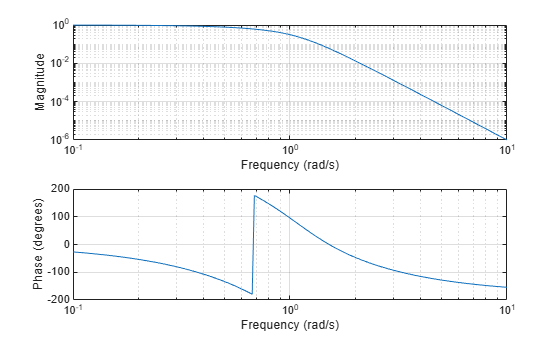Documentation

besselap

Bessel analog lowpass filter prototype

Syntax

[z,p,k] = besselap(n)

Description

[z,p,k] = besselap(n) returns the poles and gain of an order-n Bessel analog lowpass filter prototype. n must be less than or equal to 25. The function returns the poles in the length n column vector p and the gain in scalar k. z is an empty matrix because there are no zeros. The transfer function is

$H\left(s\right)=\frac{k}{\left(s-p\left(1\right)\right)\left(s-p\left(2\right)\right)\cdots \left(s-p\left(n\right)\right)}$

besselap normalizes the poles and gain so that at low frequency and high frequency the Bessel prototype is asymptotically equivalent to the Butterworth prototype of the same order . The magnitude of the filter is less than $1/\sqrt{2}$ at the unity cutoff frequency Ωc = 1.

Analog Bessel filters are characterized by a group delay that is maximally flat at zero frequency and almost constant throughout the passband. The group delay at zero frequency is

${\left(\frac{\left(2n\right)!}{{2}^{n}n!}\right)}^{1/n}$

Examples

collapse all

Design a 6th-order Bessel analog lowpass filter. Display its magnitude and phase responses.

[z,p,k] = besselap(6);        % Lowpass filter prototype
[num,den] = zp2tf(z,p,k);     % Convert to transfer function form
freqs(num,den)                % Frequency response of analog filterAlgorithms

besselap finds the filter roots from a lookup table constructed using Symbolic Math Toolbox™ software.

References

 Rabiner, L. R., and B. Gold. Theory and Application of Digital Signal Processing. Englewood Cliffs, NJ: Prentice-Hall, 1975, pp. 228–230.